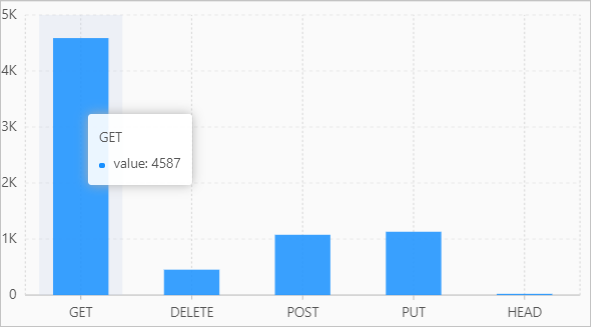In complex business scenarios, the value of a log field may be of a complex data type, such as array or map. If you want to query and analyze logs that contain fields whose values are of the preceding types, you can use an UNNEST clause to expand the field values into multiple rows for analysis.

## Syntax

• Expand an array into multiple rows. column_name specifies the column name of the rows.
``UNNEST(x) AS table_alias(column_name)``
• Expands a map into multiple rows. key_name specifies the column name of the keys and value_name specifies the column name of the values.
``unnest(y) AS table(key_name,value_name)``
Notice You can use an UNNEST clause to expand only arrays or maps. If you want to expand a string, you must convert the string to JSON data. Then, you can use the `try_cast(json_parse(array_column) as array(bigint))` syntax to convert the JSON data to an array or a map. For more information, see Data type conversion functions.

## Parameters

Parameter Description
x The value of this parameter is an array.
column_name The column name that you specify for the data expanded from the array. This column is used to store the elements in the array.
y The value of this parameter is a map.
key_name The column name that you specify for the data expanded from the map. This column is used to store the keys in the map.
value_name The column name that you specify for the data that is expanded from the map. This column is used to store the values in the map.

## Examples

### Example 1:

Expand the value of the number field into multiple rows. The field value is an array.

• Sample field
``number:[49, 50, 45, 47, 50]``
• Query statement
``````* |
SELECT
a
FROM  log,
unnest(cast(json_parse(number) AS array(bigint))) AS t(a)``````
• Query and analysis result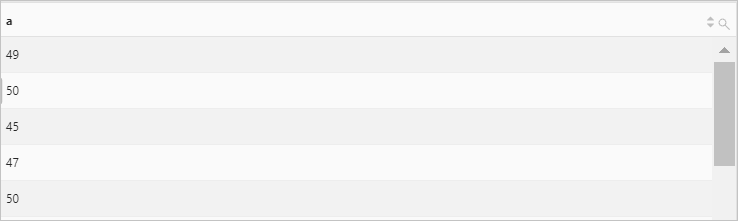### Example 2:

Expand the value of the number field into multiple rows and calculate the sum of the elements. The field value is an array.

• Sample field
``number:[49, 50, 45, 47, 50]``
• Query statement
``````* |
SELECT
sum(a) AS sum
FROM  log,
unnest(cast(json_parse(number) as array(bigint))) AS t(a)``````
• Query and analysis result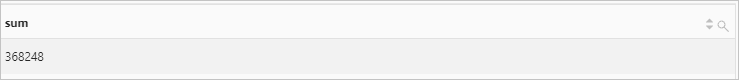### Example 3

Expand the value of the number field into multiple rows and perform the GROUP BY operation on the elements. The field value is an array.

• Sample field
``number:[49, 50, 45, 47, 50]``
• Query statement
``````* |
SELECT
a, count(*) AS count
FROM  log,
unnest(cast(json_parse(number) as array(bigint))) AS t(a) GROUP BY a``````
• Query and analysis result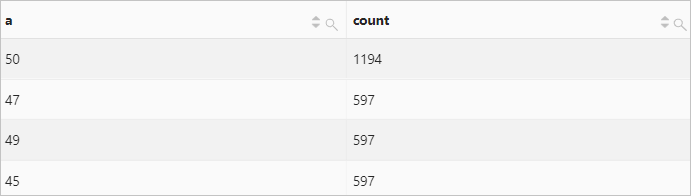### Example 4

Expand the value of the number field into multiple rows. The field value is a map.

• Sample field
``````result:{
anomaly_type:"OverThreshold"
dim_name:"request_time"
is_anomaly:true
score:1
value:"3.000000"}``````
• Query statement
``````* |
select
key,
value
FROM  log,
unnest(
try_cast(json_parse(result) as map(varchar, varchar))
) as t(key, value)``````
• Query and analysis result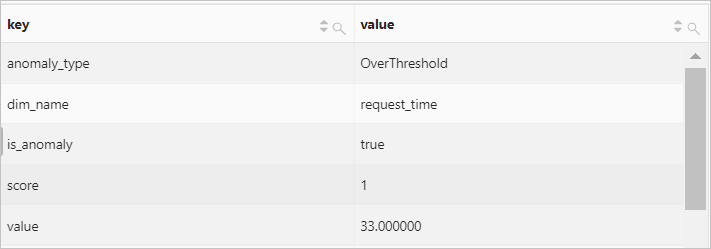### Example 5

Expand the value of the number field into multiple rows and perform the GROUP BY operation on each key. The field value is a map.

• Sample field
``````result:{
anomaly_type:"OverThreshold"
dim_name:"request_time"
is_anomaly:true
score:1
value:"3.000000"}``````
• Query statement
``````* |
select
key,
count(*) AS count
FROM  log,
unnest(
try_cast(json_parse(result) as map(varchar, varchar))
) as t(key, value)
GROUP BY
key``````
• Query and analysis result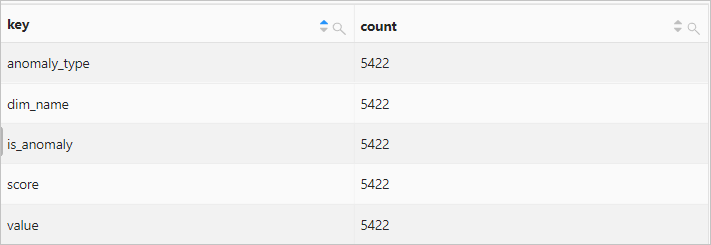### Example 6

Invoke the histogram function to obtain the number of requests that are sent by using each request method. The return value is a map. Then, use an UNNEST clause to expand the map into multiple rows and display the query and analysis result on a column chart.

• Query statement
``````* |
SELECT
key,
value
FROM(
SELECT
histogram(request_method) AS result
FROM      log
),
unnest(result) AS t(key, value)``````
• Query and analysis result